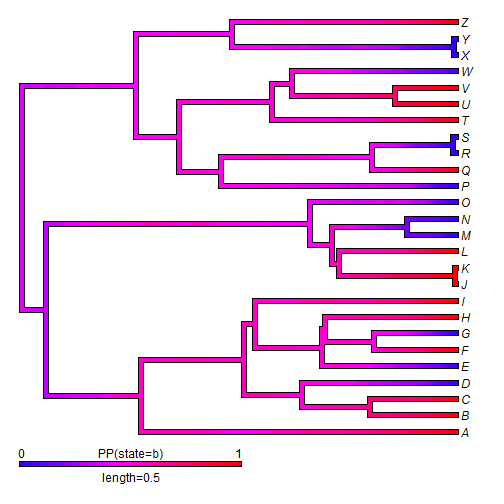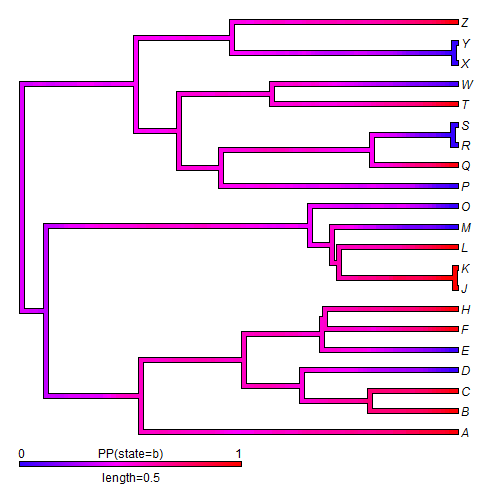## Thursday, September 3, 2015

### Bug fix in drop.tip.densityMap

A phytools user reported the following peculiar bug with the phytools function `drop.tip.densityMap`:

``````## simulate some data
library(phytools)
tree<-pbtree(n=26,tip.label=LETTERS,scale=1)
Q<-matrix(c(-1,1,1,-1),2,2,dimnames=list(letters[1:2],letters[1:2]))
x<-as.factor(sim.history(tree,Q)\$states)
``````
``````## Done simulation(s).
``````
``````x
``````
``````## A B C D E F G H I J K L M N O P Q R S T U V W X Y Z
## b b b a a b a b b b b b a a a a b a a b b b a a a b
## Levels: a b
``````
``````## now replicate the error
trees<-make.simmap(tree,x,nsim=100)
``````
``````## make.simmap is sampling character histories conditioned on the transition matrix
## Q =
``````
``````##           a         b
## a -3.440867  3.440867
## b  3.440867 -3.440867
``````
``````## (estimated using likelihood);
## and (mean) root node prior probabilities
## pi =
``````
``````##   a   b
## 0.5 0.5
``````
``````## Done.
``````
``````obj<-densityMap(trees,plot=FALSE)
``````
``````## sorry - this might take a while; please be patient
``````
``````plot(obj,lwd=4,outline=TRUE)
````````````tips<-sample(tree\$tip.label,5)
tips
``````
``````##  "I" "U" "N" "G" "V"
``````
``````obj<-drop.tip.densityMap(obj,tips)
``````
``````## x should be an object of class "contMap"
``````

I'm not sure when I introduced this bug, but it is now fixed and this will be in the next version of phytools (or if you update phytools from GitHub).

``````obj<-densityMap(trees,plot=FALSE)
``````
``````## sorry - this might take a while; please be patient
``````
``````source("../phytools/R/densityMap.R")
obj<-drop.tip.densityMap(obj,tips)
plot(obj,lwd=4,outline=TRUE)
``````That's it.